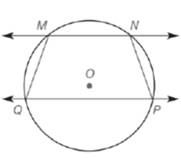Chapter 6.1, Problem 38EElementary Geometry For College St...

7th Edition
Alexander + 2 others
ISBN: 9781337614085

Solutions

Chapter
SectionElementary Geometry For College St...

7th Edition
Alexander + 2 others
ISBN: 9781337614085
Textbook Problem

If M N ↔ ∥ P Q ↔  in ⊙ O , explain why M N P Q is an isosceles trapezoid.(HINT: Draw a diagonal.)To determine

To explain:

The MNPQ is an isosceles trapezoid by using the provided figure.

Explanation

Given:

The MNPQ, in O shown below.

Postulate used:

Theorem:

The measure of an inscribed angles is equal to the one-half the measure of its intercepted arc.

If AB and BC intersect only at point, then mAB+mBC=mABC.

Calculation:

Since, M and P both are inscribed angles and NPQ and QMN are intercepted arcs.

Since, there is a theorem state that the measure of an inscribed angles is equal to the one-half the measure of its intercepted arc.

M=12(mNPQ)P=12(mQMN)

Still sussing out bartleby?

Check out a sample textbook solution.

See a sample solution

The Solution to Your Study Problems

Bartleby provides explanations to thousands of textbook problems written by our experts, many with advanced degrees!

Get Started

Find more solutions based on key concepts